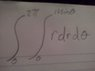# Double integral finding the area

pb23me

## Homework Statement

Use a double integral to find the area of the region bounded by the curve r= 1+sin(theta)?

## The Attempt at a Solution

I can't figure out what theta is intregrated from. I've tried from -(pi)/2 -> +(pi)/2 and that doesn't work. I've also tried from 0-> 2(pi) and that doesn't work. I have no clue what I am supposed to integrate theta to. I would really appreciate some help with this.

Staff Emeritus
Homework Helper
Gold Member

## Homework Statement

Use a double integral to find the area of the region bounded by the curve r= 1+sin(theta)?

## The Attempt at a Solution

I can't figure out what theta is integrated from. I've tried from -(pi)/2 -> +(pi)/2 and that doesn't work. I've also tried from 0-> 2(pi) and that doesn't work. I have no clue what I'm supposed to integrate theta to. I would really appreciate some help with this.
Have you done a polar plot?

0 → 2π should give the right answer.

What does your integral look like ?

pb23me
i put a picture up

#### Attachments

•IMG00072-20120408-2058.jpg
13.6 KB · Views: 969
Staff Emeritus
Homework Helper
Gold Member
i put a picture up
$\displaystyle \int_0^{2\pi}\int_0^{1+\sin(\theta)}r\,dr\,d\theta$​

What do you get for tour final answer?

pb23me
I get (3(pi) + 2)/2

Staff Emeritus
Oh I see what I've been doing now. When I evaluated theta at 0 I was getting -2. I forgot to multiply this by 1/2 which would have given me 3(pi)(-2+2)/2 or 3(pi)/2 for my final answer. Thank you so much for all of your help I was stuck on that problem for awhile.